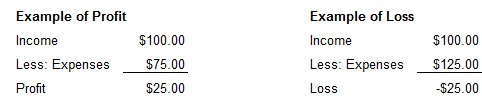# Java Program Calculate Profit and Loss | Java Programs

Java program to find profit and loss – Here we written simple Java code to find profit & loss along with detailed algorithmic explanation & sample output as well. If you have any queries you can leave a comment.

Profit and loss is a concept which is not exclusive to business owners but it is something that everyone is ought to know to be financially wise. Want to sell an old merchandise at an online selling portal like Craigslist or Olx? Or even selling your unused guitar that you bought last summer, to a friend of yours? You ought to learn “Profit and Loss” buddy!

As you might already know, every transaction has a cost price, a selling price, or a profit or loss depending on the transaction.  If the cost price is more than the selling price, then the transaction is bound to be a loss and vice versa.

Here are the basic concepts of Profit and Loss

Cost price – The amount incurred to us while manufacturing or buying that commodity.

Selling price – The amount of money received after selling a given commodity.

Profit – The amount one gains in transaction when the selling price is greater than the cost price.

Loss – The amount one loses in a transaction when the cost price was greater than the selling price.Profit and loss Formulas –

Profit = SP – CP

Loss = CP – SP

Profit percentage = (Profit /Cost Price) x 100

Loss percentage = (Loss / Cost price) x 100

## Java Code For Profit & Loss

For writing any code, following certain systematic steps makes it easy.

First is to understand problem statement, then look for any mentioned constraints, then focus on inputs followed by determining the expected output and finally decide the logic or solution.

Here, our problem statement is to find the profit or loss of a certain product.

Our input values are two different prices of the product- one is the cost price while the other is the selling price.

Since money can be whole number or decimal we make use of double datatype. Our output is either the amount of profit or amount of loss based on the input.

Now, focusing on the solution, we need to first gather the our inputs.

We make use of the Scanner class to read these inputs at runtime. We first create object referencing to the Scanner class and then using this, we invoke the nextDouble() method to read our input (cp,sp).

With all the inputs in hand, there are three possible scenario. They are as follows:

If the cost price (cp) is greater than the selling price (sp) or if cp-sp>0 then, it is a loss and the amount lost is (cp-sp). This will be the output under this case which can be displayed on the console screen.

If the selling price (sp) is greater than the cost price (cp) of if cp-sp<0 then, it is profit. The amount gained would be (sp-cp). This is the output under this scenario which will be displayed on the console screen.

Else when both of this is not the case, when both cost price (cp) and selling price (sp) are equal then, it is NEUTRAL. It is neither a profit nor a loss.

else

System.out.println(“NEUTRAL”);

Output:

x

## Plus Star Pattern Java Program | Patterns

Java program to print plus star pattern program – We have written the below print/draw ...